# Grid-based Path Finding

In this tutorial we will make a path finding simulation based on a 2-dimensional array grid. This will give us the basic idea in creating path finding activities to our games. We will visualize the grid using empty square objects as tiles and a round object as our avatar that can move on that grid area with our command.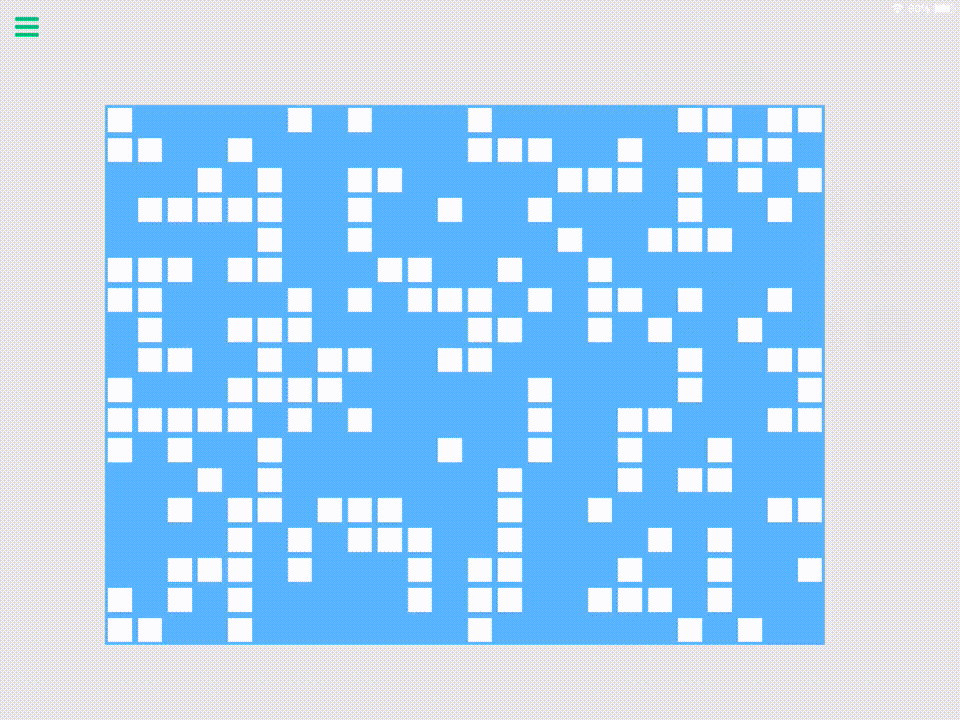I. Setting up the objects

We will only use four objects in our project. Our green avatar, a blue tile, a white blockade, and the grid label for setting up and visualizing our grid.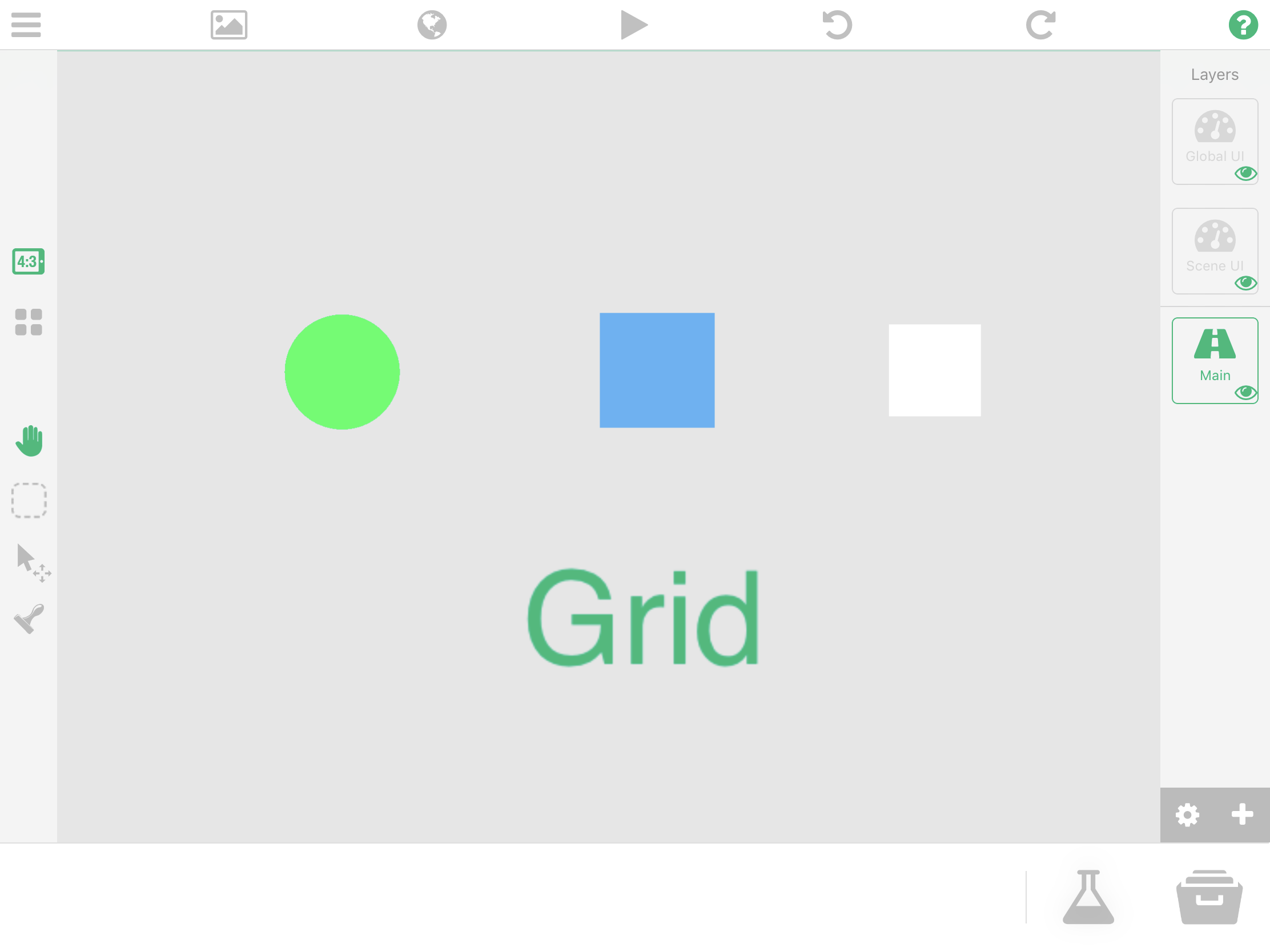Avatar

Our avatar is an empty objects that has an x and y scale of 50%. Have its collision set to a round shape so that it would look like the one in the image below. This object will have three predefined attributes: on tile, previous tile, and target tile. Set these attributes' default values to zero so that they won't have a null value when we to try to fetch them later on.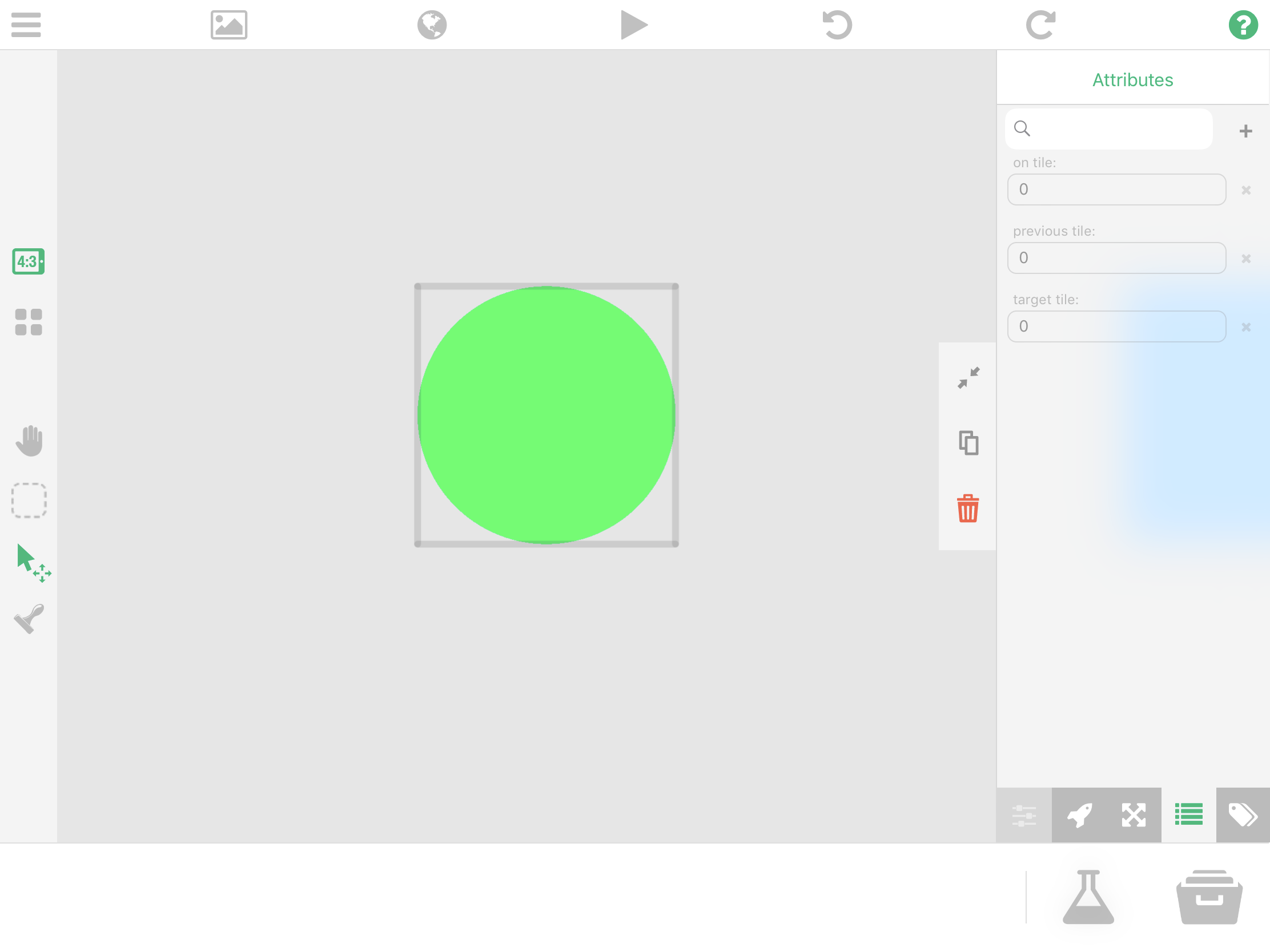Tile Object

Create another empty object that will be used to visualize the tiles in our grid. Its scale is also 50% and has only one predefined attribute: on tile. Set also this value to zero.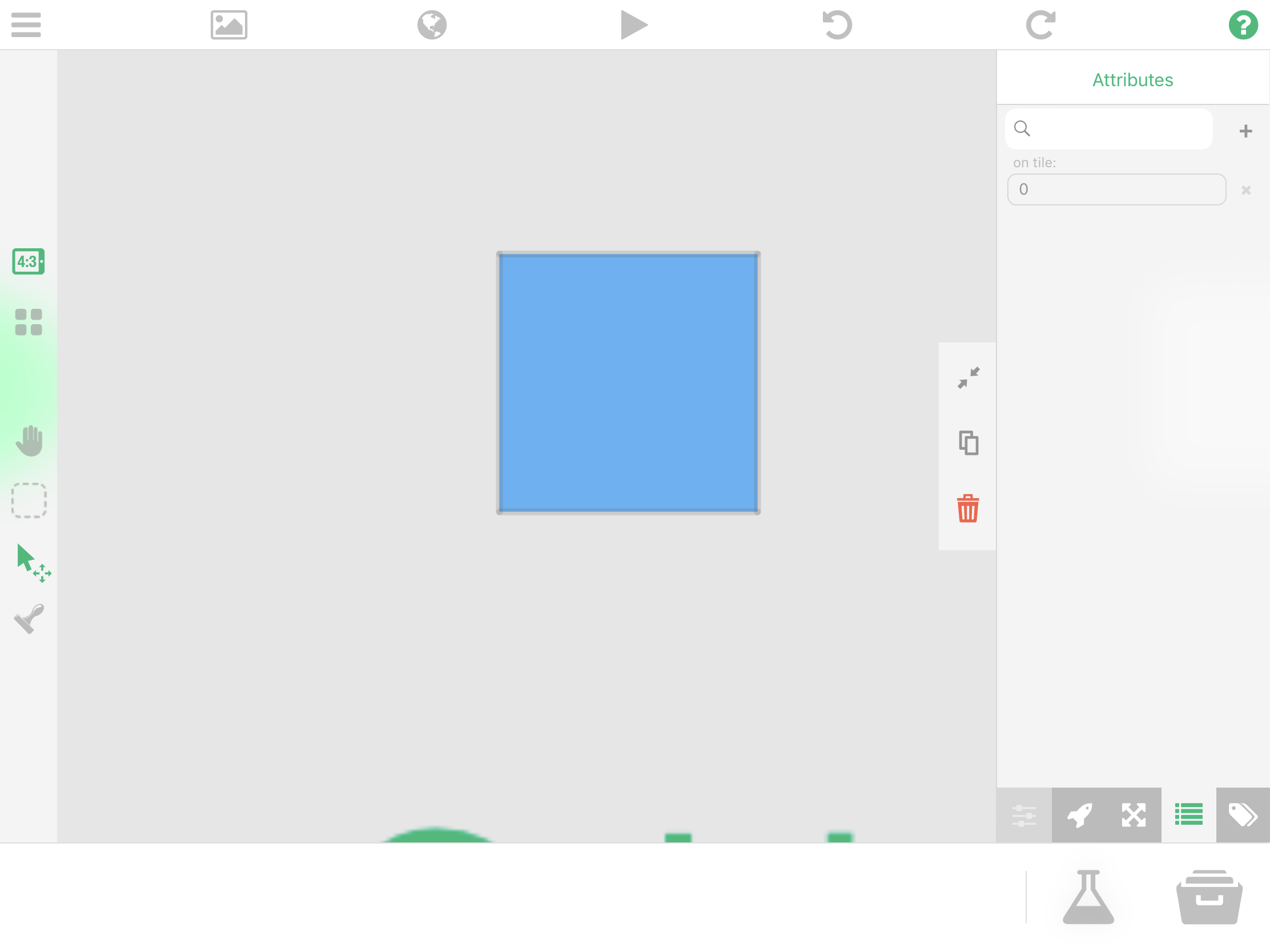Create another empty object that will serve as blockades in our grid. This is a white empty object with a scale of 40%. Our avatar will not be able to traverse to a tile that has a blockade on top of it.

Grid Label

This is just a label that will have the behaviors that will allow the setting up and visualization of our grid. It has predefined attributes: start x with a value of 4, and start y with a value of 3. These values are offsets that will be used as a references when spawning our tiles in order to position our grid neatly to the center.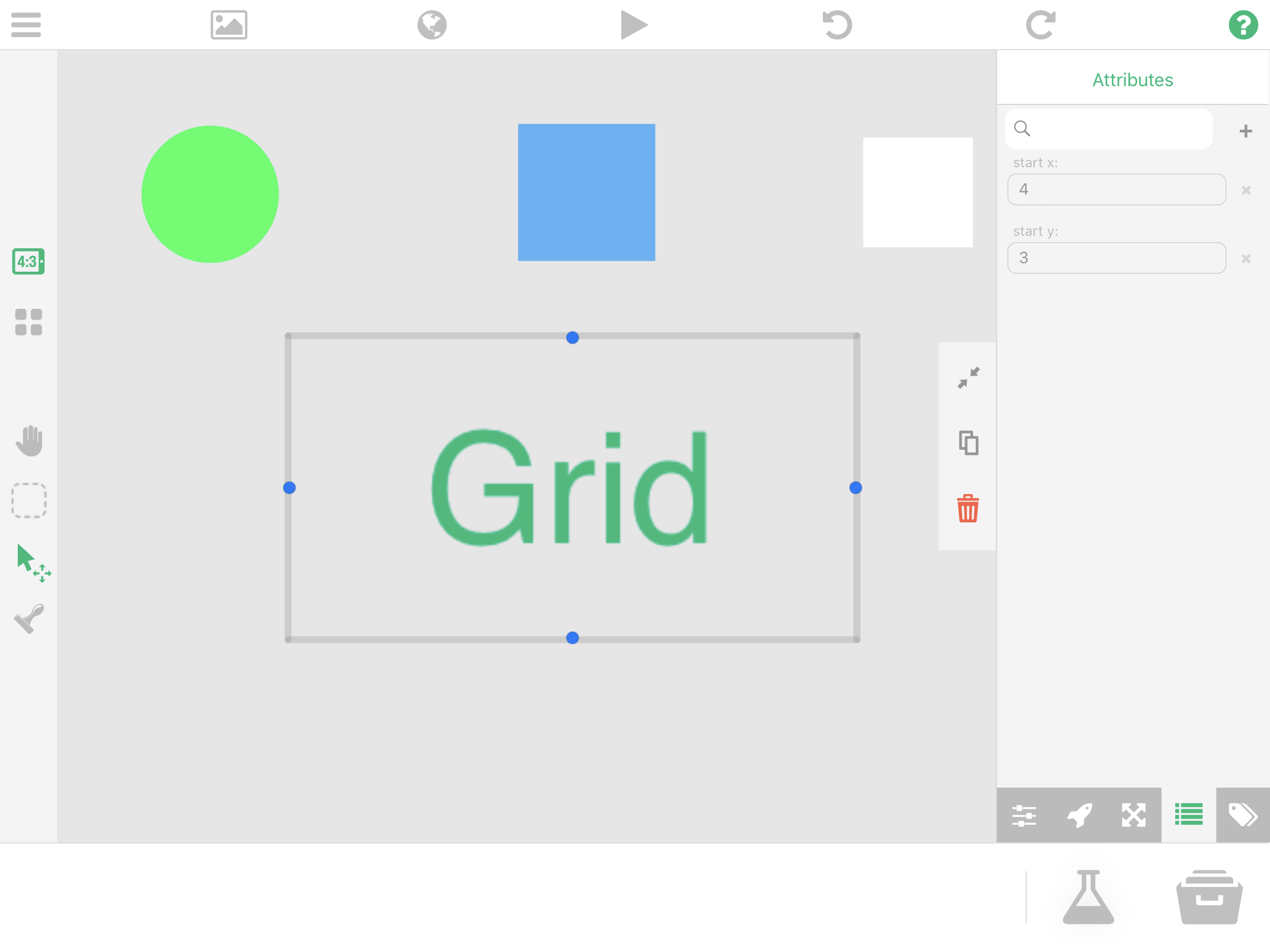Avatar/Tile Predefined attributes:

We define a tile here as a 2-sized array which will have an x and y value based on our grid.

• on tile - this is the tile value that our object is currently on in our grid
• previous tile - a tile that was previously traversed by our avatar
• target tile - the tile that our avatar will go to

II.  Setting up the grid

Inside the grid label is where we will define our 2D array and the spawning of our grid. There are 2 main key points in this object: setting the array, and visualizing the array. Let's do first the set array bundle.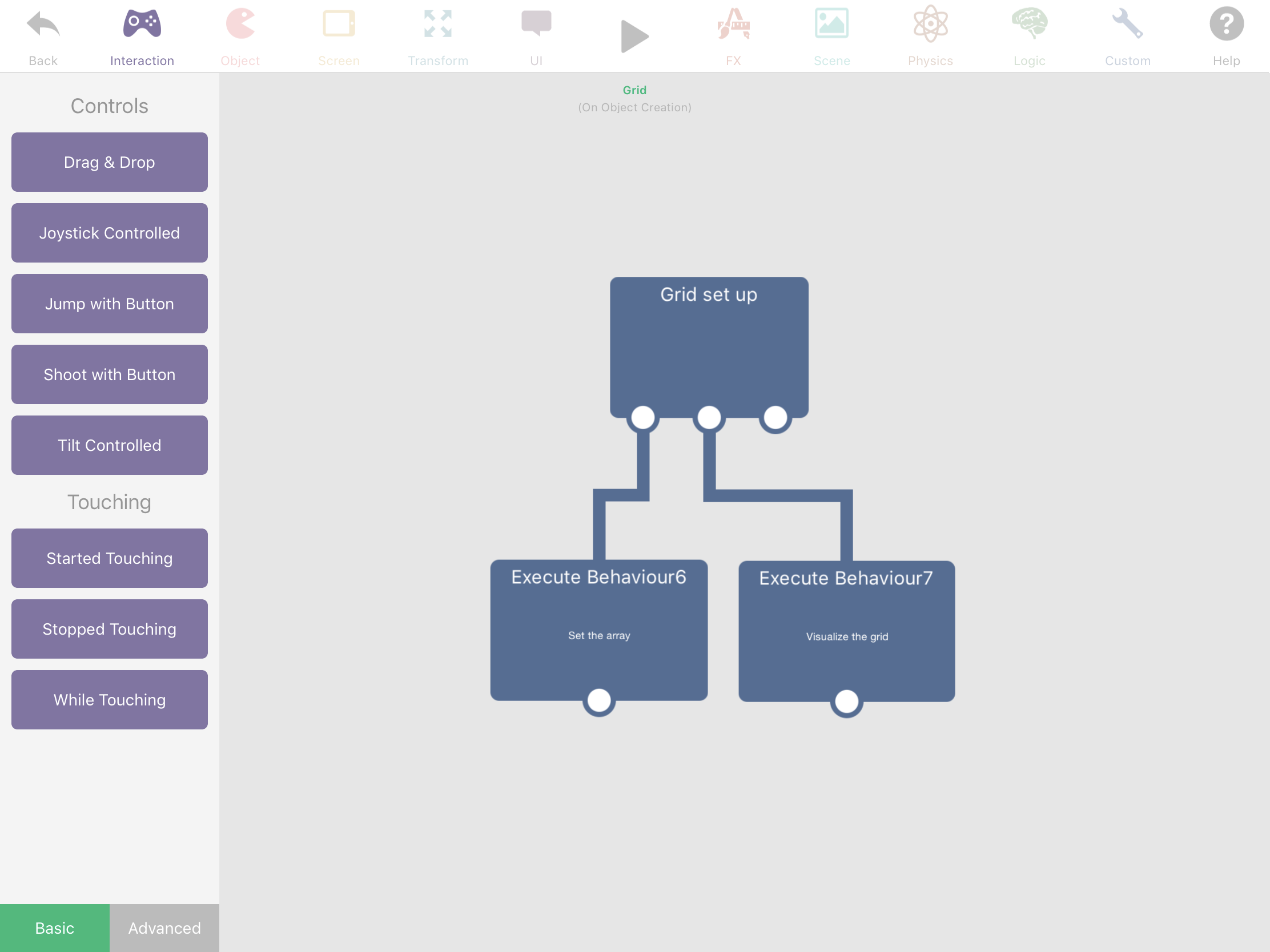Set the array - bundle

First is to create an empty array named 'Grid'. This array will contain our 2 dimensional array. We will have a box container named 'x row count' that has a value of 24 and another empty array named 'y row' which has 18 empty values.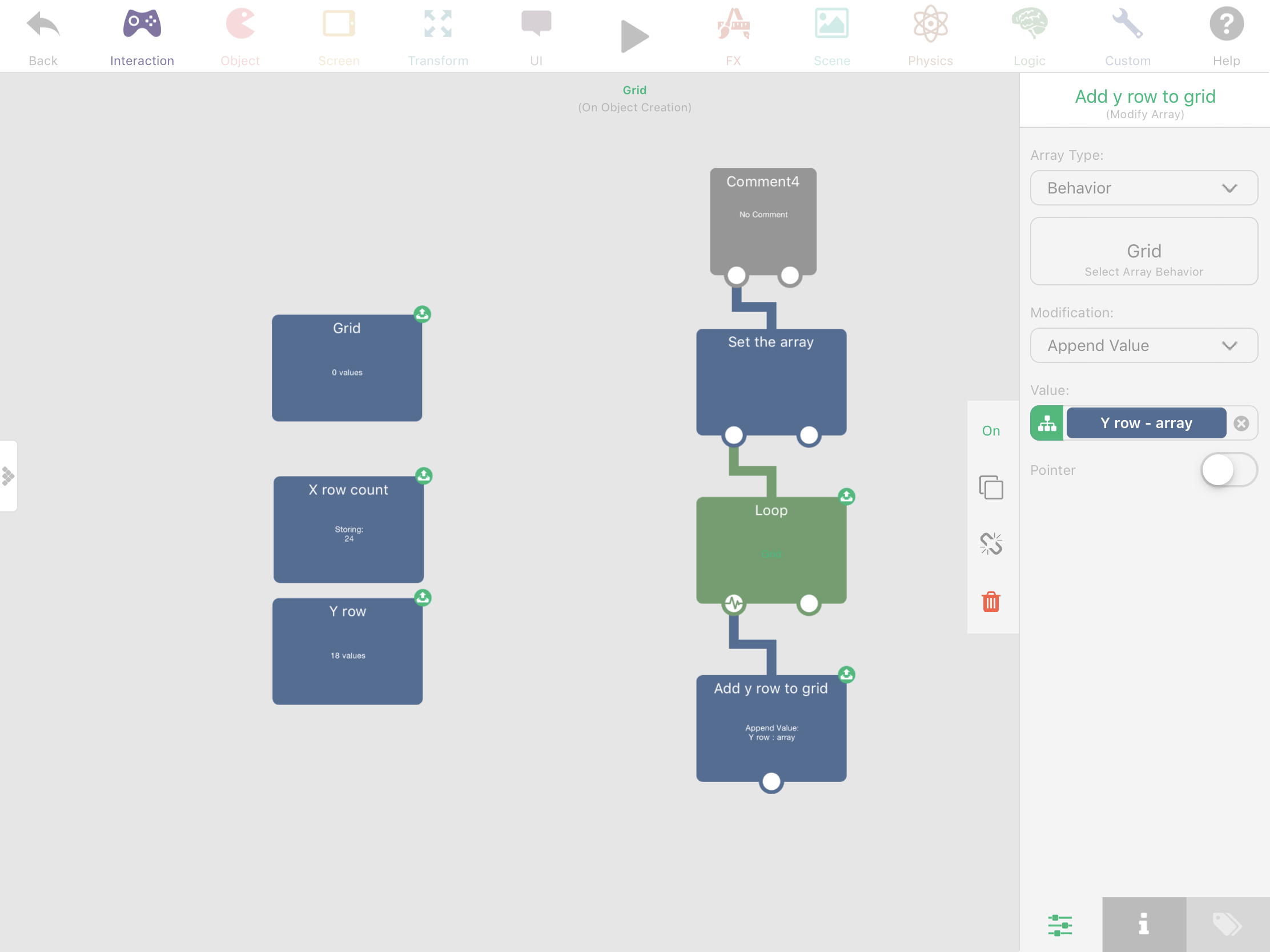The first behavior in our bundle is a loop with a repetition times of 'x row count'.  For each step of this loop, we will add an empty y row to the 'Grid array'. This will create an array within an array with a dimension of 24x18.

Notice that we've connected an inactive comment at the start of our bundle to negate the automatic execution of this root behavior. A root behavior is a behavior that does not trigger based on any event and is placed openly in the interface.

Visualize the grid - bundle

The main thing that we will do here is to traverse on each value of our 'Grid array' and spawn a tile object. We will also add a condition in our loop that will allow spawning of a white blockade on top of the tile object.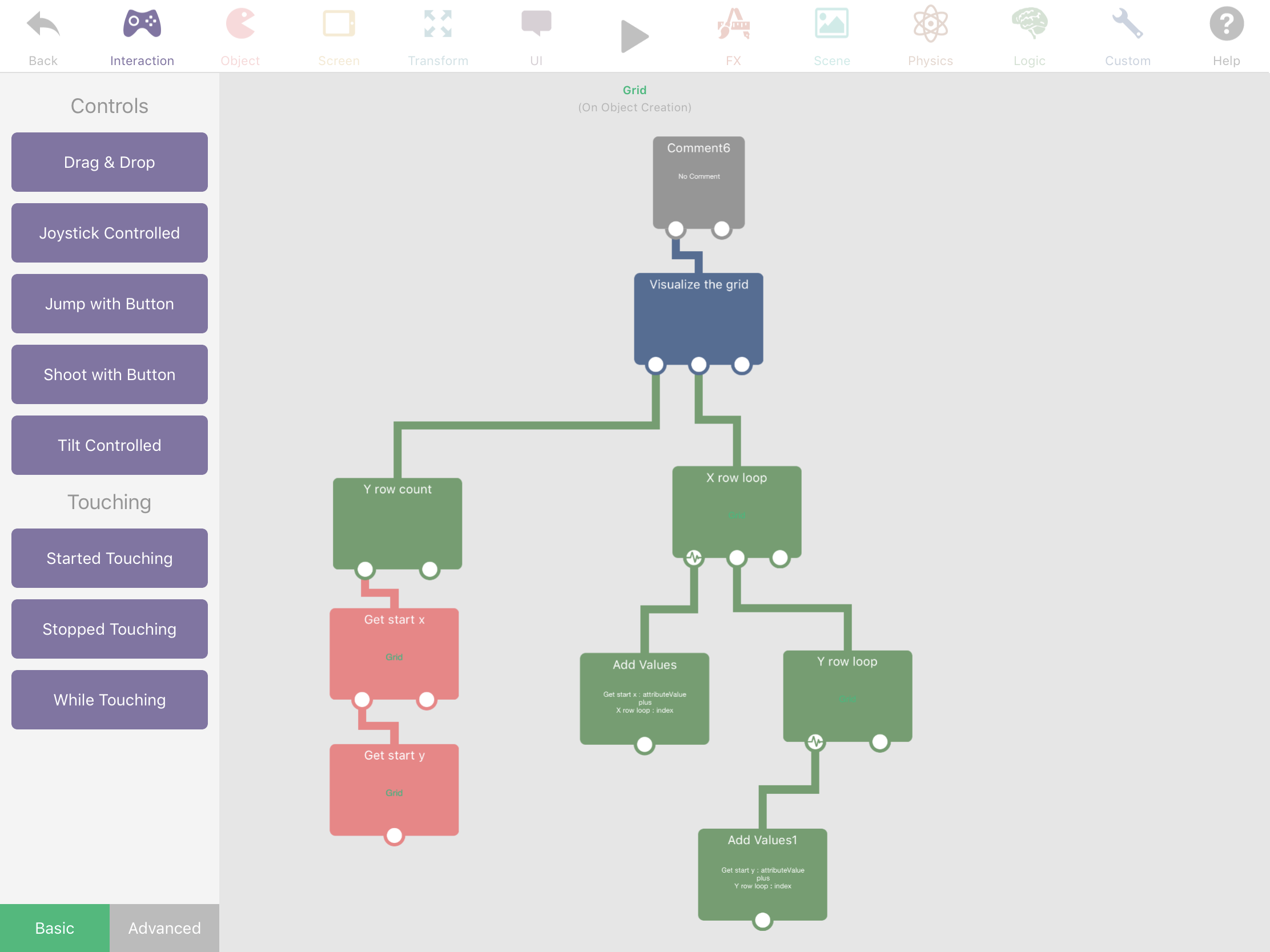First is to get the array count of our 'y row array', the 'start x' attribute value of this object and the 'start y'.

Create a loop that has a repetition of 'x row count' and for each step of this loop, we will add the index value of that loop and the 'start x' attribute value. This results to an absolute x position that we will use to position our spawned tiles later on. Next is the 'y row loop' that has a repetition of 'y row count'. For each step of this loop, we will add the index value of that loop and the 'start y' attribute value, which will now result to the absolute y position.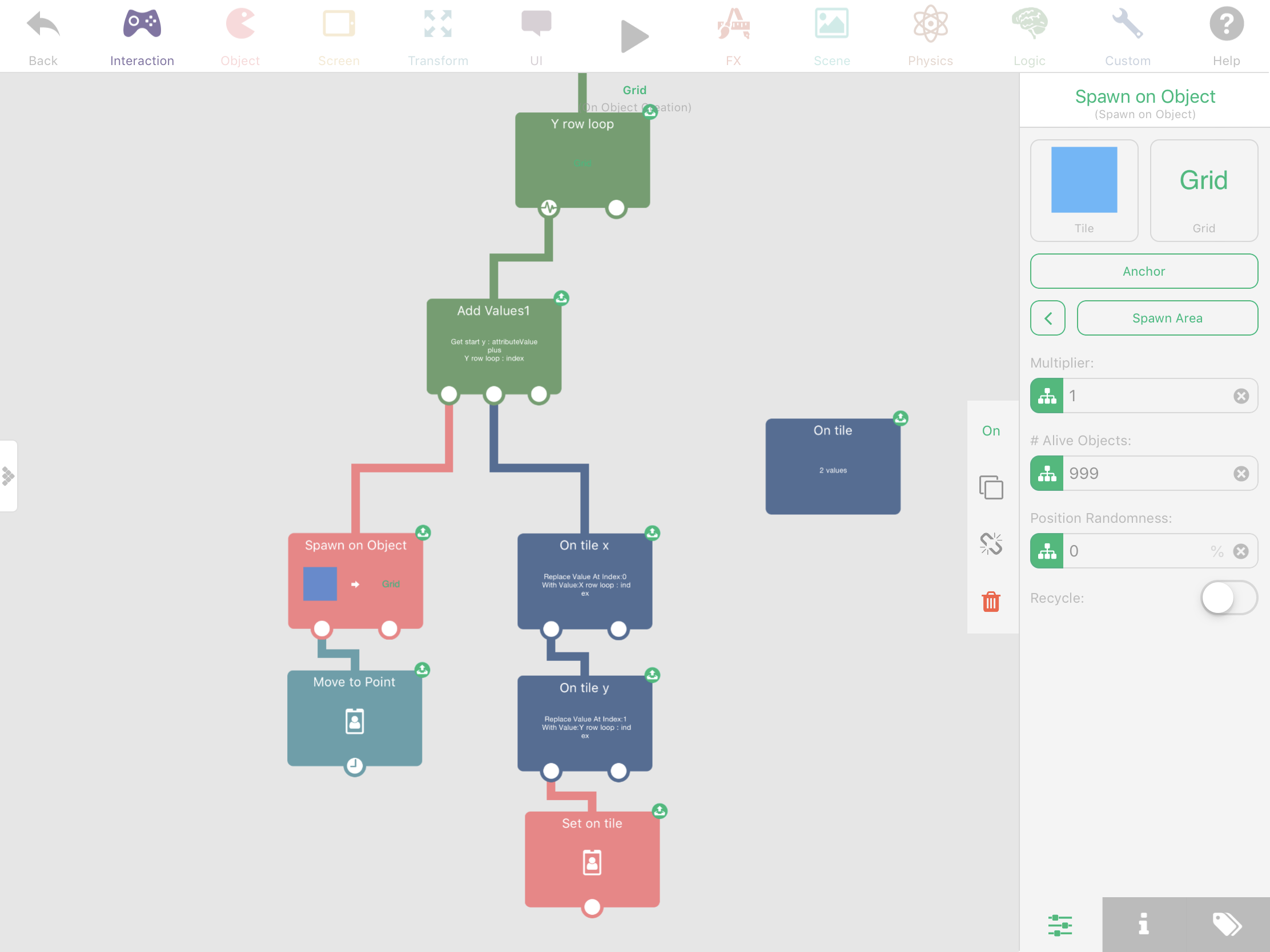After adding the values under the 'y row loop' is to spawn the blue object. Set the #of alive objects of that to 999 because we will need a bunch of them for our grid, and also with a duration of 0. Then move that spawned object to an x point with a value from the x loop 'add values', and the y point of value from the y loop 'add values'.

Next is to add an array with 2 empty values named 'On tile' which we will only use as an array placeholder. After moving the tile object is to modify the 'On tile' array by replacing its value at index 0 with value of 'x row loop'. Then add another array modification that, replaces the value at index 1 with 'y row index'. This is the x and y coordinates of our grid that the object will have as an 'on tile' attribute.

Now, set the attribute of the spawned object with a dynamic key, 'on tile', and with a value of 'On tile - array'.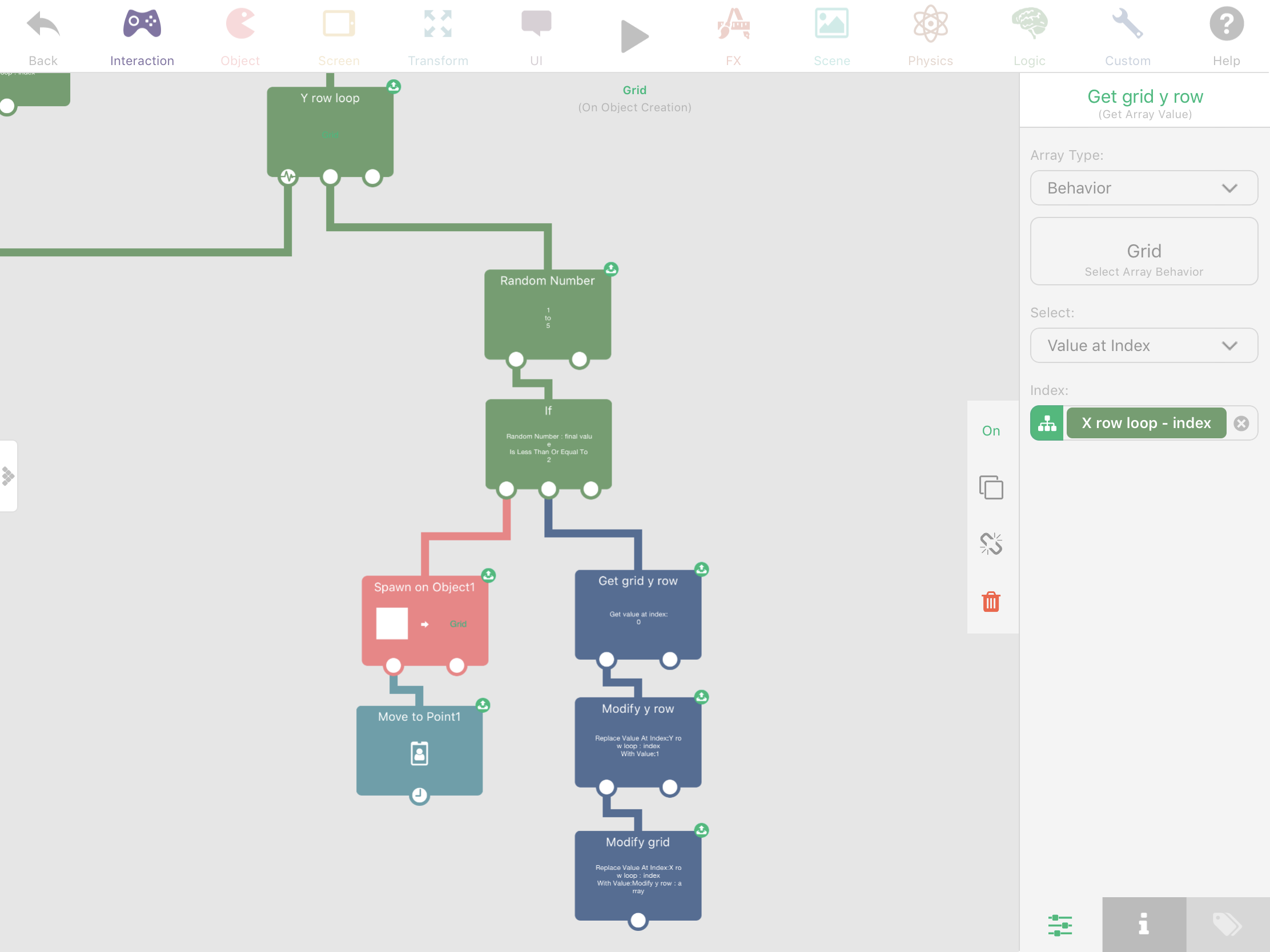Still, under the y row loop, is the condition of spawning the white object blockade. We will have a 2 out of 5 chance of executing this bundle which will add to the randomization of our grid. Then we will flag a value of 1 to the x and y coordinate of that in our 'Grid' array which tells us that that coordinate has a spawned blockade.

First is to have a random number of 1 to 5. If that number is less than or equal to 2, we will spawn the blockade. Set its #Alive objects to 999 and move that object to the same point as the blue tile that we spawned before, and also with a duration of 0.

Next is to store the information that this coordinate was occupied by a blockade, if it was spawned. We do this by flagging that coordinate with a value of 1. First is to get the value at index 'x row loop' of the 'Grid' array. This value will give as an array of a 'y row'. We will modify this 'y row' by replacing its value at index 'y row loop' with value 1, which is the flagged value. Because this value modification only applies to that value, and not to the 'Grid itself', we still have to modify the 'Grid' array. Replace the value at index 'x row loop' of 'Grid' array with a value of 'modify y row - array'.

Once played, it should result to this kind of form.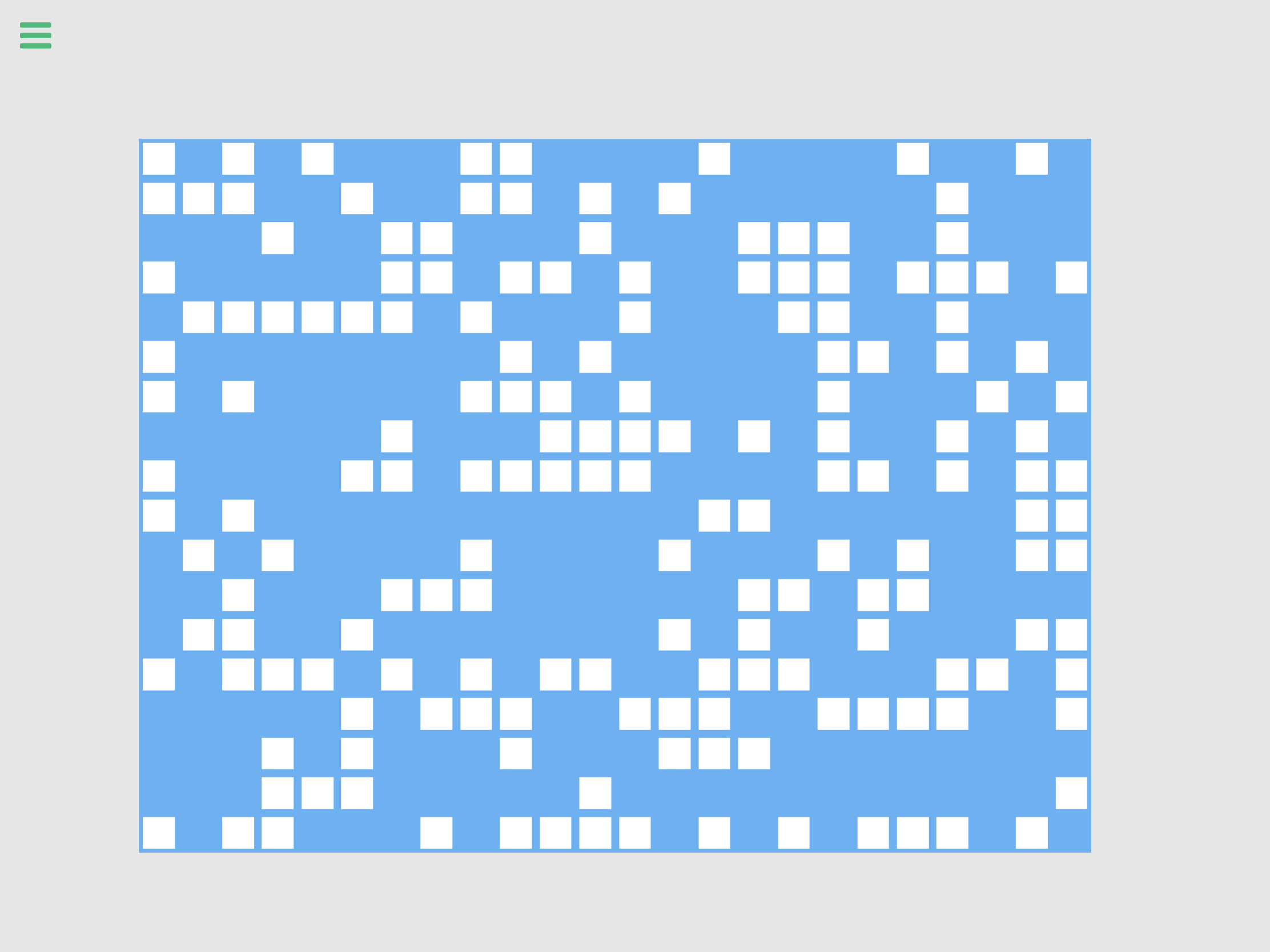III. On Tile Press

To be able to interact with the grid itself, we will need a bundle behavior whenever we press a blue tile. The first press will result to the appearance of our avatar in our grid, and any successive press after that event will start its path finding.

Inside the blue object is a behavior bundle that has 3 main points: is to reset the previous tile of our avatar, set its on tile/set its target tile, then set a color animation to visualize the touch event on screen.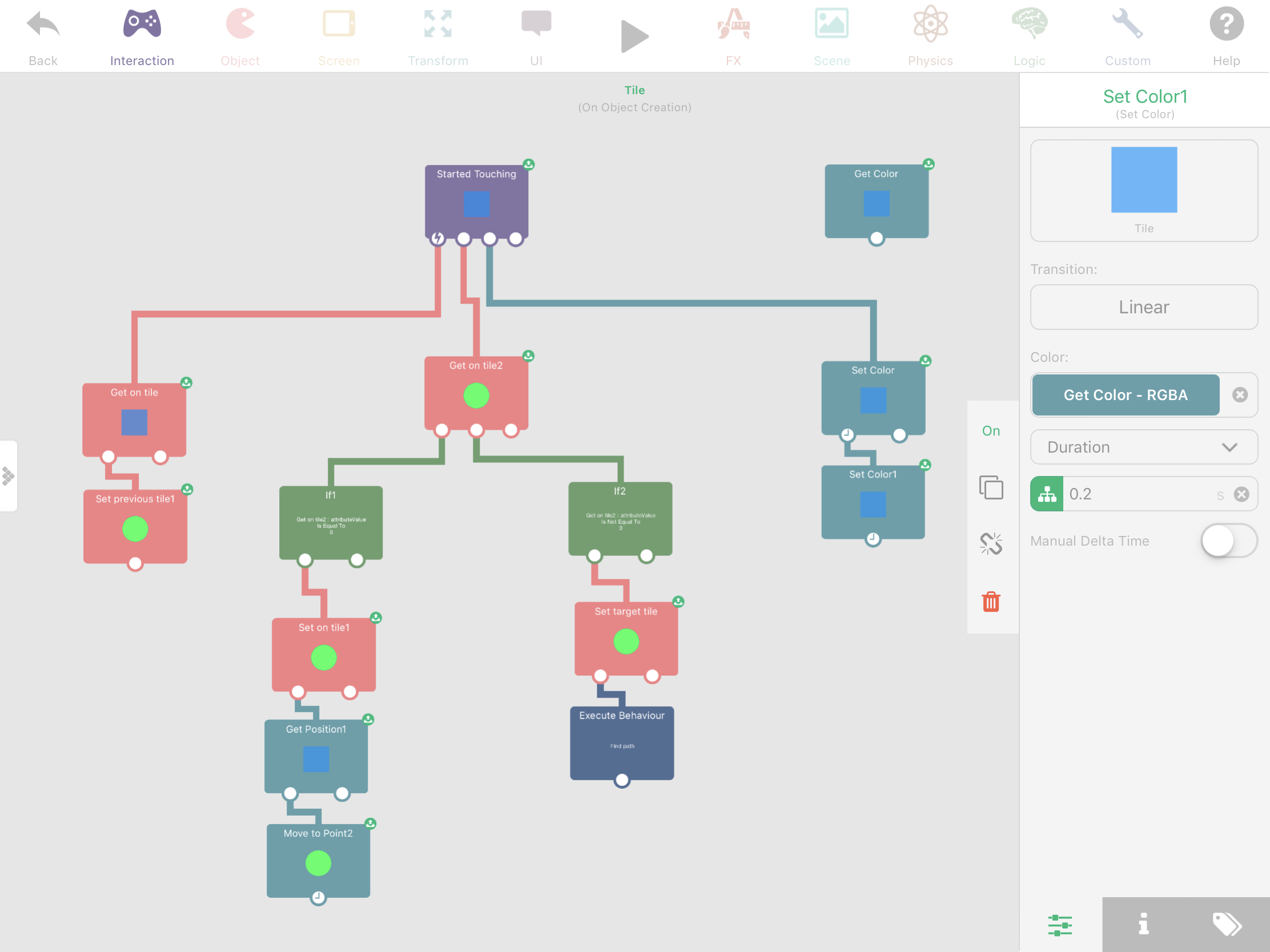First is to get the 'on tile' attribute of the tile object. Set the 'previous tile' attribute of our avatar to 0. Our avatar will be set to avoid going back to a 'previous tile' that's why we reset the 'previous tile' on every tile press.

Next is to get the 'on tile' attribute of our avatar. The first if condition is whether the 'on tile' value is equal to zero. This means that if the avatar has not appeared yet, then this will result to true. If its true, the we set its 'on tile' attribute with a value of the tile object's 'on tile' attribute. Then move our avatar's position by getting the position of the tile object, pointing its x and y values to that point with a duration of 0.

If the 'on tile' value is not equal to zero. we will set the 'target tile' attribute of our avatar with the tile object's 'on tile' attribute value. This means that our avatar is already in our grid, and then, we can execute the behavior bundle, 'find path'. We still don't have this behavior yet but we will fix this again after doing the later part of this tutorial for the 'find path' bundle.

Now that we have properly set our avatar to our grid, we'll just have to visualize this press event. First is to have a root behavior 'get color' to get its original color. Then after the press event, we will set the color of the tile object to black with a duration of 0, then set its color back again to its original value with a duration of 0.2.

Pressing on a blue tile should result to this.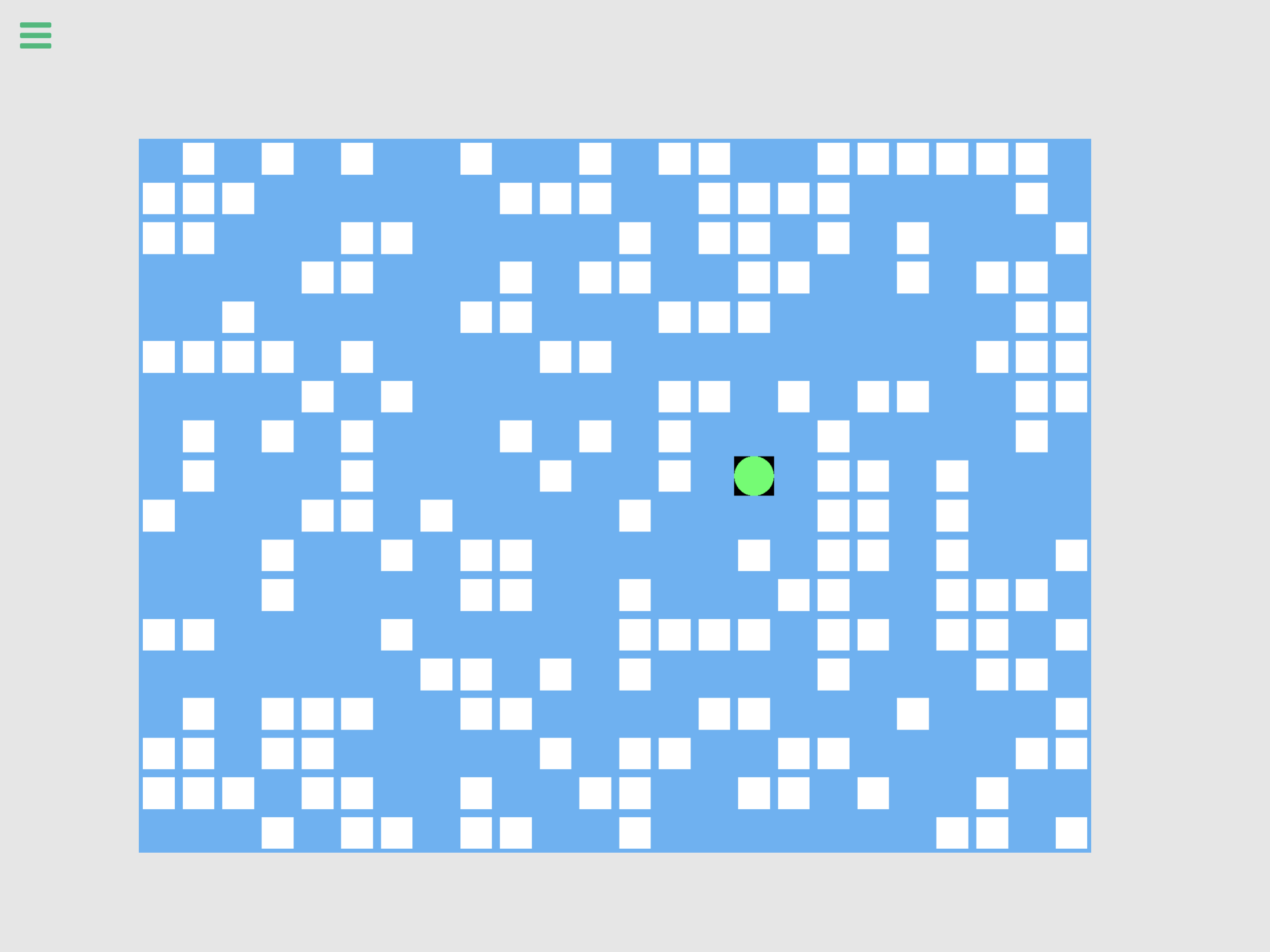IV. Execute Find Path Bundle

For this last part of our tutorial, we will do the algorithm that allows us to find the closest tile that our avatar can take to reach its destination. This bundle will be executed repeatedly for every tile step that our avatar moves on our grid.

We will set up the algorithm inside our avatar object and that will have three main points: finding of adjacent tiles, finding the nearest adjacent tile, and the movement to that adjacent tile.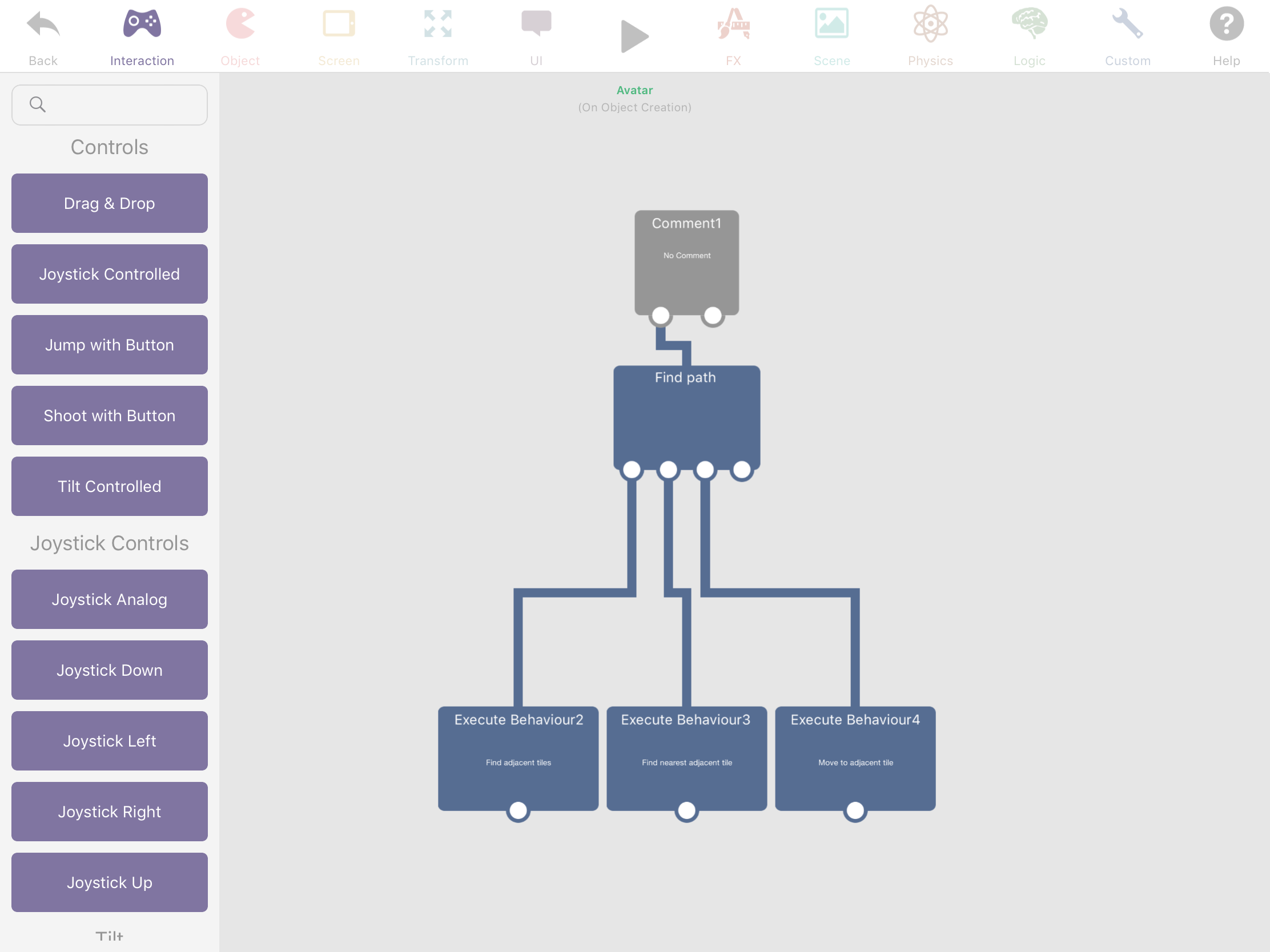Finding the adjacent tiles

In this part, we will collect all the adjacent tiles that our avatar would be able to traverse to. We will check its four directional adjacent tiles and see if those tiles has a blockade on top of it or if it was previously stepped on.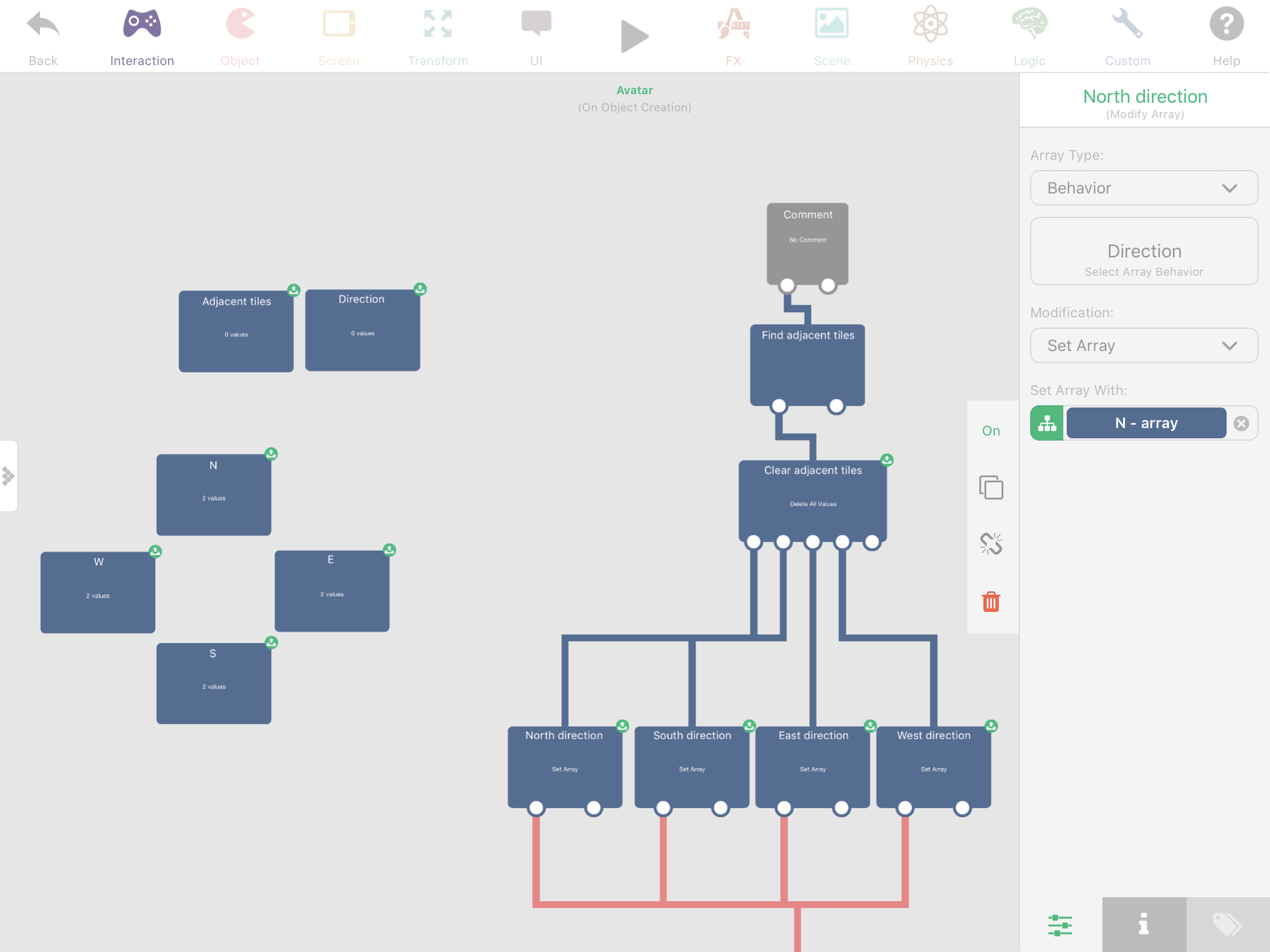First is to add our 'Adjacent tiles' array. A 'Direction' array with 2 empty values, and the four directional arrays that has 2 values for each: N (0,1), S (0,-1), E (1,0), W( -1,0).

The first behavior in our bundle is to clear our adjacent tiles. Because we will do this bundle repeatedly, we need to have our collected 'adjacent tiles' reset each time. The next four behaviors are modifications to the 'Direction' array. We set the array of 'Direction' array four times with the four directional arrays, then execute the bundle below. We do this to create a loop with different 'Direction' array values each time.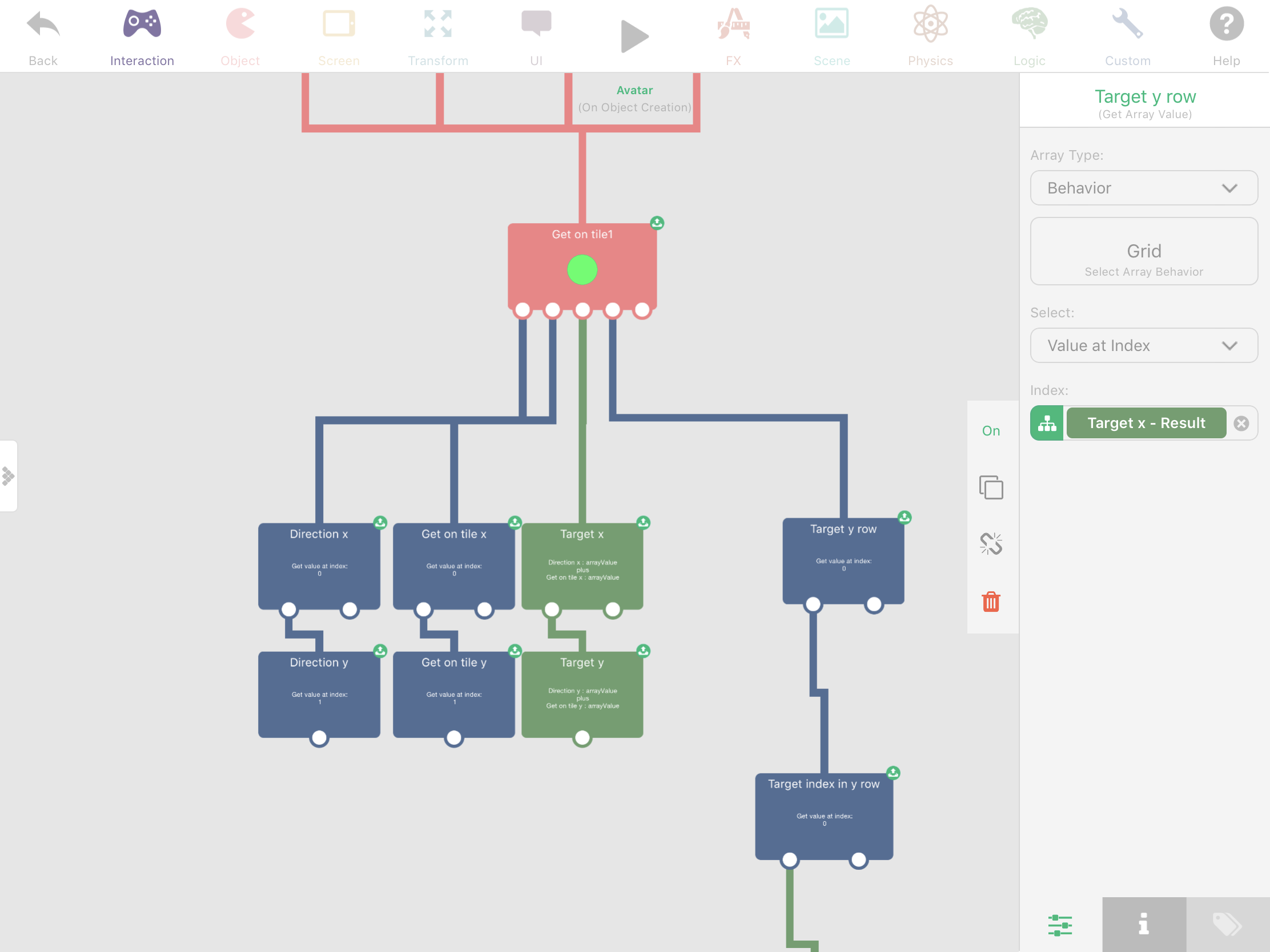Now that we have the direction, we only have to combine their x and y values with the 'on tile' attribute of our avatar. Then we'll be able to get our target value in the 'Grid' array to know if that tile was flagged with a blockade.

'Direction x' is the value of 'Direction' array at index 0, 'get on tile' x is the value of 'get on tile' at index 0. Target x is the additive values of 'direction x' and 'get on tile x'. Same goes with y values but with a target index of 1.

'Target y row' is the array value of 'Grid' array at index 'Target x'. And then the 'Target index in y row' will be the value of 'Target y row' array at index of 'Target y'. That is the coordinate in our grid that will be able to tell us if it was flagged with a blockade.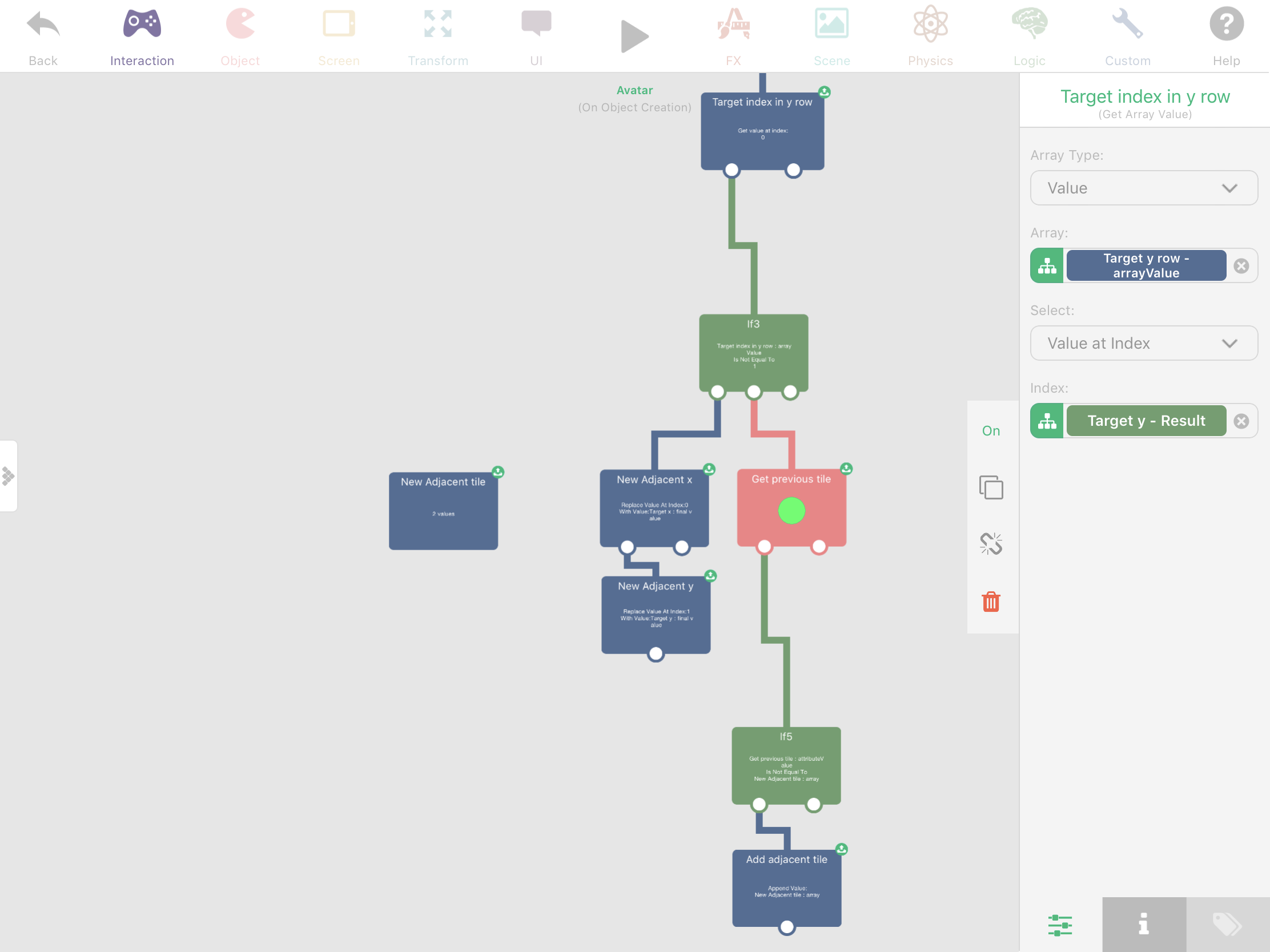If the value in 'Target index in y row' is 1, then that target tile was flagged as blocked. But if the value is not equal to 1, then we can continue in our bundle.

Because our Target x and y passed our condition, we've added a new empty tile array named 'New adjacent tile'. This will store the values of Target x and y as a tile array. We will set the value of Target x to the index 0 of 'New adjacent tile' and Target y to it's index 1.

Now get the 'previous tile' attribute of our avatar. If the 'previous tile' is not equal with the 'new adjacent tile', that would mean that the new tile was not previously traversed. Then, we can safely add the 'New Adjacent tile' to the 'Adjacent tiles' array by appending it. We will set the value of 'previous tile' later on after successfully moving our avatar to another tile.

"You can expand this bundle by making an 8 directional arrays which allows your avatar to move to diagonal directions."

Finding the nearest adjacent tile

Now that we have collected our reliable adjacent tiles, we just need to get the adjacent tile that is nearest to our avatar's target tile. We will do the calculation by using a distance formula supplied by the x and y values of our target tile and the adjacent tiles.

There are two key points in this bundle: the initialization of variables for calculation, and the calculation of tile distances inside a loop.

a. Initialization of variables

First is to get the array count of our adjacent tiles. Then we get the 'target tile' attribute of our avatar and get its x and y values. We will add 2 new box containers: the 'Min distance' and the 'Target tile index'. We set the initial value of 'Min distance' to 9999, and the value of 'Target tile index' to 0. The min distance is set to a high number because we need the least distance from the start. The target tile index is our reference index in the 'Adjacent tiles' array that has the least distance to our 'target tile'.

Our main goal here is to get the index of the tile inside our 'Adjacent tiles' array that is nearest to our 'target tile'.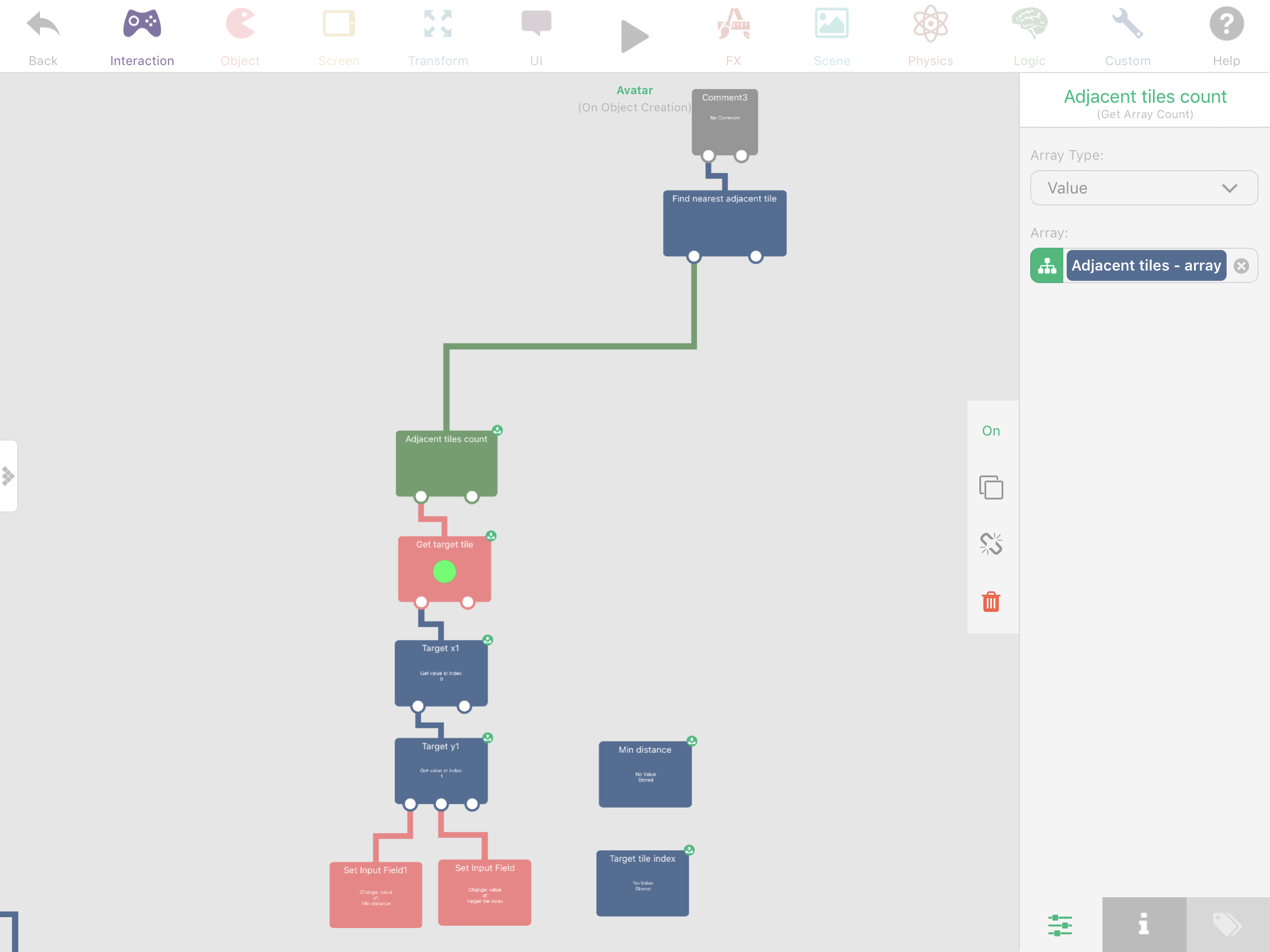b. Calculation of tiles distances

In this bundle, we will do the calculation inside a loop that we will do for each adjacent tile. The loop repeats by 'adjacent tiles count' and we will get the tile value of the 'Adjacent tiles' array using the index of that loop.

Get the x and y values of that adjacent tile and then we'll get the distance between our 'target tile' and the 'adjacent tile' using this formula:

`distance = sqrt((x2 - x1)^2+(y2 - y1)^2)x1 = Target xx2 = Adjacent xy1 = Target yy2 = Adjacent y`

We first subtract their x and y values, multiply it to itself to get its exponential values, add those values, and then square root the added values, to get the distance value.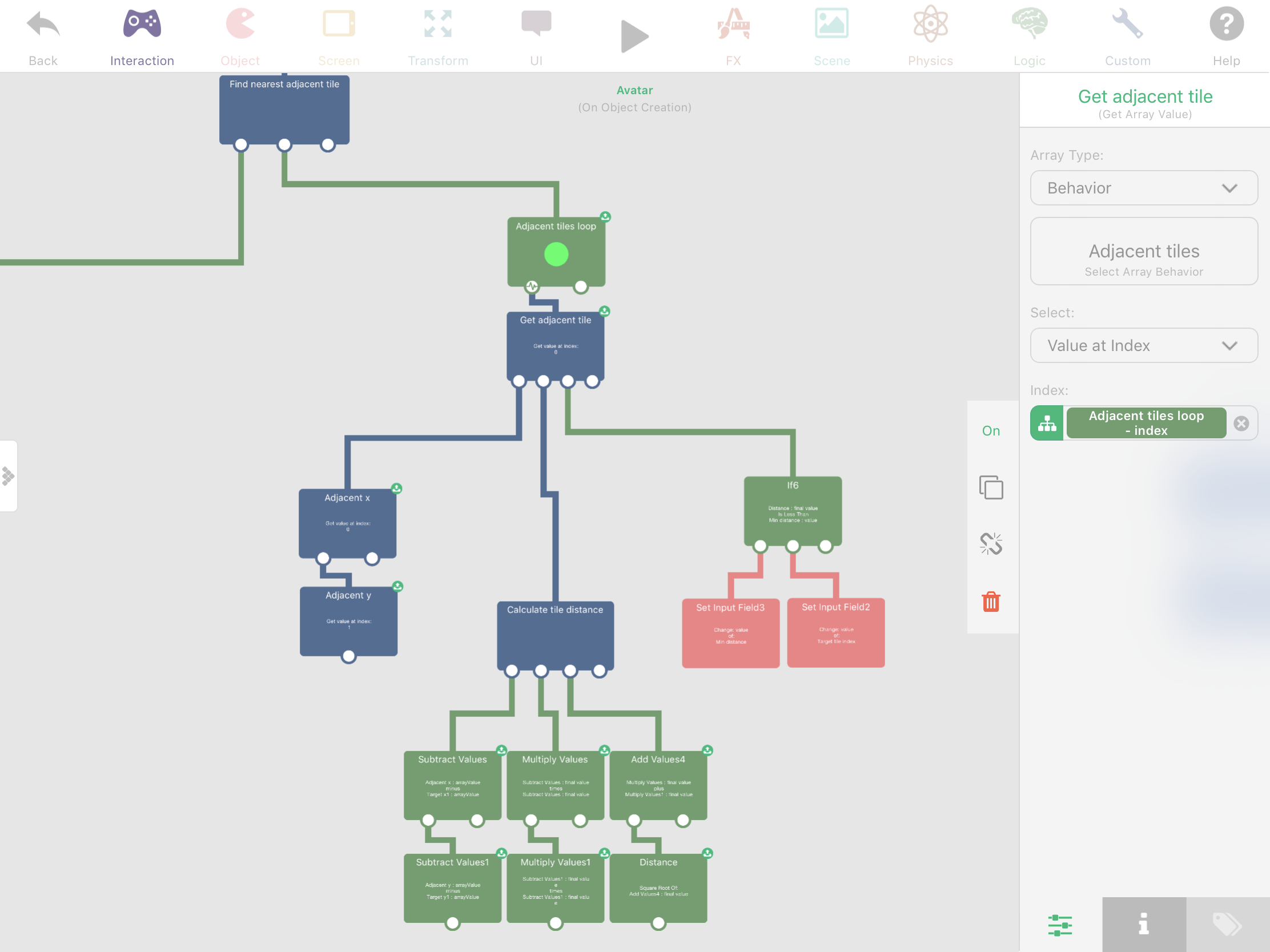If the 'Distance' value is less than the 'Min distance' box container value, we will then set the 'target tile index' value to the current index of the loop, which is the index of our fetched tile inside the 'Adjacent tiles' array. We also set the 'Min distance' box container value with the 'Distance' value for us to compare it with the next tile of our loop.

After the end of the loop, we have compared each one of our adjacent tiles and we've got the index value of the adjacent tile that is nearest to our 'target tile'.

Movement to the adjacent tile

In this last bundle of our tutorial, we will move our avatar to the nearest adjacent tile and redo the whole 'Find Path' bundle if we haven't already moved to our 'target tile'.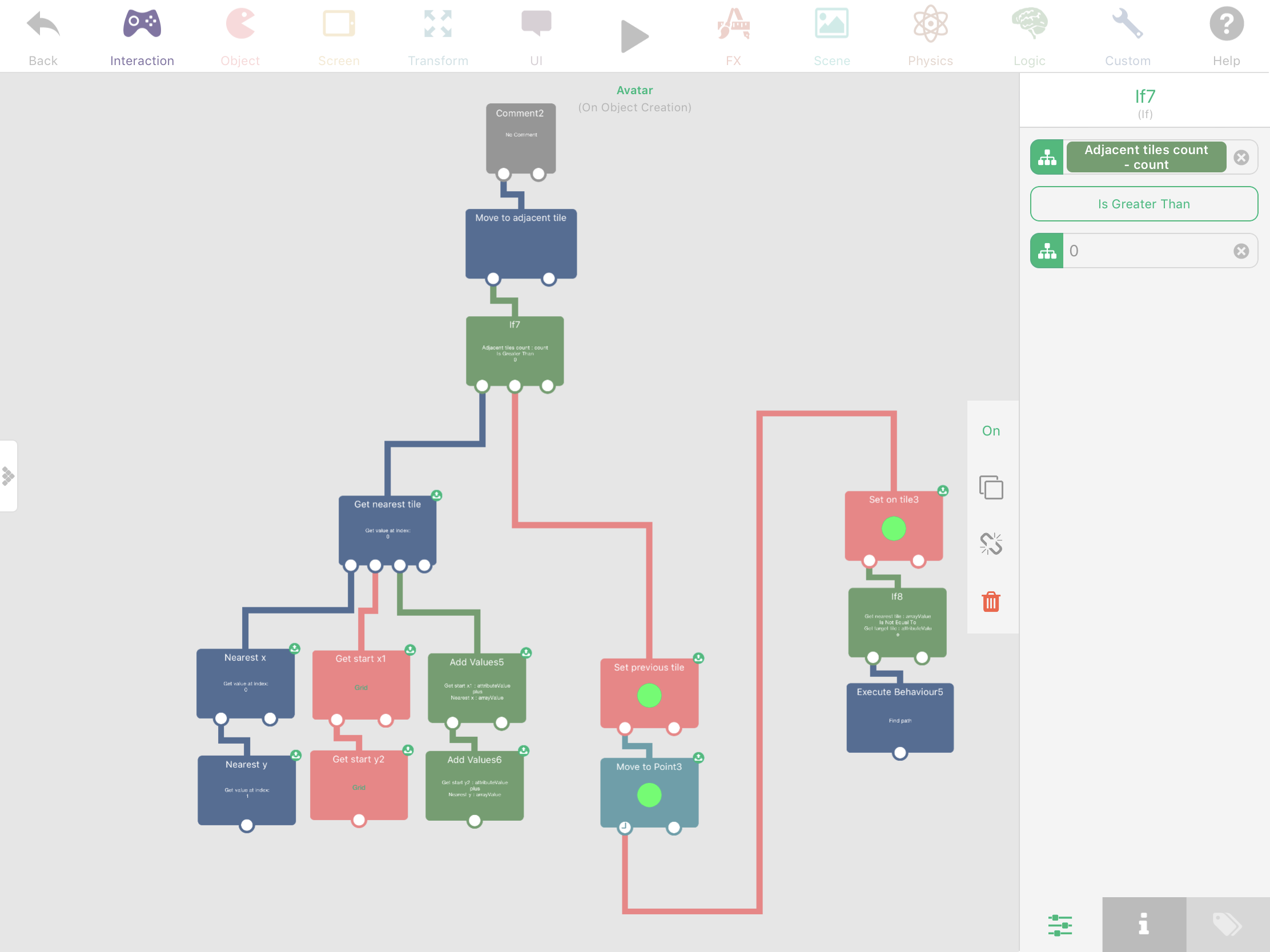The first thing is to check if our 'Adjacent tiles count' is greater than 0. This is to ensure that will only move if we fetched at least reliable adjacent tile.

Then we 'Get the nearest tile' by using our 'Target tile index' value from the previous bundle to get the array value of the 'Adjacent tiles' array.

In order to get the exact point where our avatar will move to, we will need  the x and y tile values of that tile, and also get the 'start x' and 'start y' attribute values of our 'Grid' label. We then add the x value of our 'nearest tile' and 'start x' value, and also the same with the y values. These added values are our target x and y points where our avatar will move to.

Before we move to that point, we set our avatar's 'previous tile' with its current 'on tile' attribute value. We can get this 'on tile' value from the previous bundles. We then move our avatar to our target points, with a duration of 0.2.

After the completion of the 'Move to point' behavior, we now set the 'on tile' attribute value of our avatar with the 'Get nearest tile' array value. If the 'Get nearest tile' array value is not equal to our' target tile', we will then execute the 'Find Path' bundle, thus, redoing the whole bundle again. The 'target tile' attribute value can be get from the previous bundle.

By pressing on a any blue tile, and after the appearance of our avatar on the grid, the 'Find Path' bundle will be executed. This will trigger the movement of the avatar from tile to tile.

Conclusion

In this tutorial, we have learned to make an object path finding activity using an array that we called the 'Grid', which is used store an information whether that tile was occupied or not. You can expand this idea, by using object ids as information storage instead of just a digit number for our 'Grid' array. This will give us more reliable information that we can use when doing path finding. For example, we can get the attributes of that object id to know if it is alive or not, whether it will allow us to step on its tile or not.

This tutorial only served the basic idea behind path finding. One drawback from this is that it does not create the shortest path possible to a target tile, and only checks its current adjacent tile. This can result to a movement loop that does not reach anywhere.

However, this can be solved by expanding our path finding algorithm. We can do this by reiterating on our fetched adjacent tile to get its adjacent tiles too until we find our target tile. Each found nearest adjacent tile will be added to a list. In order to prevent an infinite loop, add a condition that if that adjacent tile was already checked, that tile won't be added to our list. Also, add a max limit on the iteration count to prevent an infinite loop which is caused by the target tile if it is too far or not possible to be found. Once that iteration finds the target tile, we then exit that iteration and start moving the avatar from tile to tile using the tile list.